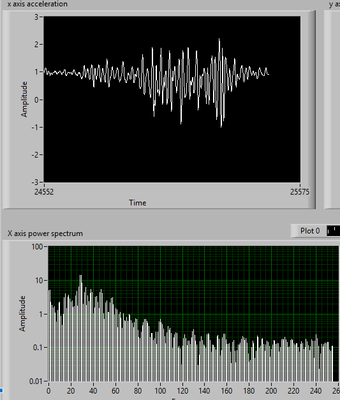LabVIEW

cancel
Showing results for
Did you mean:

Plotting FFT for vibrations

Hello!

I am trying to do FFT on the signal from accelerometer. I have an array of 512 that i need to plot repeatable. I tried using a Express VI for Power Spectrum or plotting real part of of FFT or the modulus showing on x-axis the nr. of samples/2.First graph is plotting acceleration in g-units, the second is power spectrum on logarithmic scale.

I want to plot the power spectrum by frequency for vibration analysis, knowing that sample rate is 200Hz.

Can anyone help me with some ideas. Thanks in advance.

Message 1 of 8
(1,247 Views)

Re: Plotting FFT for vibrations

Attach your VI so we can see how you are doing it.

You might have to apply some scaling to the X axis.  Some FFT functions are purely mathematical and don't take in things like the amplitude of the signal or its frequency.  You may have to factor in the sampling rate along with the number of samples.

Message 2 of 8
(1,215 Views)

Re: Plotting FFT for vibrations

I attached the VI. I take data from accelerometer via serial, and store it in 3 arrays. Now, I'm trying to plot FFT for vibration analysis for the 3 axes.

Tried with expressVI, plotting real part or plotting modulus value, but there's no input for calculating frequency on horizontal axis.

Message 3 of 8
(1,209 Views)

Re: Plotting FFT for vibrations

Seeing the actual VI tells me a lot.

The problem is that you can't get an actual frequency is because your incoming data doesn't have any sample rate associated with it.

The Spectral Measurements Express VI's want a waveform as the data type on the Signals input.  You are feeding it an array.  Array has no sample rate.  A waveform does in that one of its cluster values is dT or the delta Time between samples.  So your array gets coerced into a waveform (see the red dot on the Express VI).  It gives it a default dT value of 1.

If you build your array into a Waveform and give it the real dT value, then your Express VI's should show the frequency values.

Message 4 of 8
(1,198 Views)

Re: Plotting FFT for vibrations

I have inserted a build waveform block before Spectral Measurements block with dt=0.005 and the values on horizontal axis are from 0 to 1.2 which is still incorrect.

I am missing something, but I don't know what.

Message 5 of 8
(1,196 Views)

Re: Plotting FFT for vibrations

What WOULD be the correct values then?

You are getting data from a Serial device?  What is this device?

Message 6 of 8
(1,188 Views)

Re: Plotting FFT for vibrations

Well, I need to compute FFT on an 1D array with 256 values. Values are from -4 to 4 and represents acceleration. My sample rate is 200Hz(i read the ADC every 5ms). I tried to build a waveform like you said but the values on frequency axis are wrong.(tested with a sine of 50 Hz )

Message 7 of 8
(1,180 Views)

Re: Plotting FFT for vibrations

Attach a VI where the data you recorded is saved as default in a control.  Also show a screenshot of the results.

It is impossible to debug a problem from words alone.

Message 8 of 8
(1,171 Views)